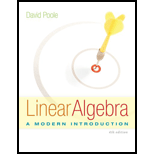## Solutions for Linear Algebra: A Modern Introduction

### Book Details

David Poole's innovative LINEAR ALGEBRA: A MODERN INTRODUCTION, 4e emphasizes a vectors approach and better prepares students to make the transition from computational to theoretical mathematics. Balancing theory and applications, the book is written in a conversational style and combines a traditional presentation with a focus on student-centered learning. Theoretical, computational, and applied topics are presented in a flexible yet integrated way. Stressing geometric understanding before computational techniques, vectors and vector geometry are introduced early to help students visualize concepts and develop mathematical maturity for abstract thinking. Additionally, the book includes ample applications drawn from a variety of disciplines, which reinforce the fact that linear algebra is a valuable tool for modeling real-life problems.

## Sample Solutions for this Textbook

We offer sample solutions for Linear Algebra: A Modern Introduction homework problems. See examples below:

## More Editions of This Book

Corresponding editions of this textbook are also available below:

Linear Algebra A Modern Introduction David Poole Fourth Edition 2015
4th Edition
ISBN: 9781305016958
LINEAR ALGEBRA: MODERN INTRO
4th Edition
ISBN: 9780357539262